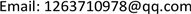1. 引言

x i 3 − 1 = 0 ,     i = 1 , 2 , ⋯ , n (1.1)

x i 2 + x i x j + x j 2 = 0 (1.2)

2. 预备知识

2.1. 升列的定义

1) c l ( F ) < c l ( G ) 。

2) c l ( F ) = c l ( G ) = p > 0 ，但 deg x p ( F ) < deg x p ( G ) 。

A : A 1 , A 2 , ⋯ , A r

1) r = 1 , A 1 ≠ 0 。

2) r > 1 , 0 < c l ( A 1 ) < c l ( A 2 ) < ⋯ < c l ( A r ) ，且对任意 j > i , A j r e d / A i 。

2.2. 矛盾列的定义

2.3. 基列的定义

B : B 1 , B 2 , ⋯ , B s

1) 存在 j ≤ min ( r , s ) ，使得 A 1 ~ B 1 , ⋯ , A j − 1 ~ B j − 1 ，但 A j ≻ B j 。

2) s > r 且 A 1 ~ B 1 , ⋯ , A r ~ B r 。

2.4. 特征列的定义

2.5. 多项式组的零点分解

3. 主要结论3.1. 吴方法解决染色问题的过程

x i 2 + x i x j + x j 2 , ( i , j ) ∈ { ( 1 , 2 ) , ( 1 , 5 ) , ( 1 , 6 ) , ( 2 , 3 ) , ( 2 , 4 ) , ( 2 , 8 ) , ( 3 , 4 ) ,       ( 3 , 8 ) , ( 4 , 5 ) , ( 4 , 7 ) , ( 5 , 6 ) , ( 5 , 7 ) , ( 6 , 7 ) , ( 7 , 8 ) }

C S = { x 1 − x 7 , x 2 + x 7 + x 8 , x 3 − x 7 , x 4 − x 8 , x 5 + x 7 + x 8 ,                   x 6 − x 8 , x 7 2 + x 7 x 8 + x 8 2 , x 8 3 − 1 }

z e r o ( P S ) = z e r o ( C S / J ) + z e r o ( P S , I 1 ) + ⋯ + z e r o ( P S , I 8 )

3.2. 吴方法和Groebner基方法在解决染色问题时的比较

Groebner基方法 在计算过程中会使多项式对增加很快，选取多项式对的无序性也会造成结果不同，项序的选择和终止条件的复杂性与否都会影响计算的效率。吴方法 在计算多项式方程组时效率较高，速度也很快，整体来说在解决染色问题上吴方法比Groebner基方法更快速和有效。